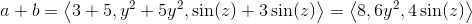# Calculus 3 : Vector Addition

## Example Questions

### Example Question #31 : Vector Addition

Find the sum of the vectors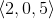and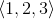.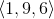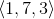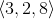Explanation:

The formula for adding vectors is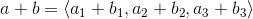.

Plugging in the values we were given, we get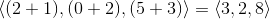### Example Question #32 : Vector Addition

What is the sum of the vectors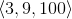and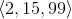.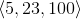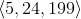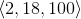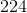Explanation:

The formula for adding vectors is.

Plugging in the values we were given, we get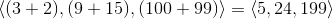### Example Question #33 : Vector Addition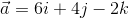and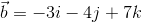. Find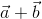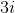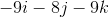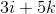Explanation: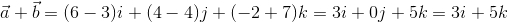### Example Question #34 : Vector Addition

Find the sum,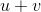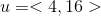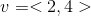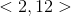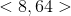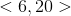Explanation:

The sum of two vectors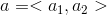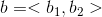is defined as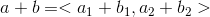For the vectors in this problem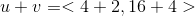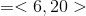### Example Question #35 : Vector Addition

Perform the matrix operation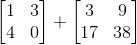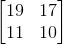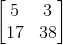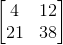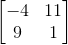Explanation:

To find the sum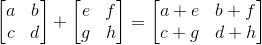. Using the matrices in the problem statement, we get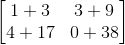=### Example Question #36 : Vector Addition

Solve: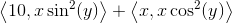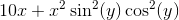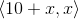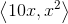Explanation: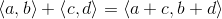Note that our final answer is a vector.

### Example Question #37 : Vector Addition

Solve: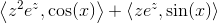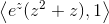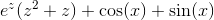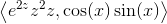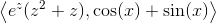Explanation:

The sum of two vectors is the vector given by the sums of the respective components (for example,).### Example Question #38 : Vector Addition

Perform the following vector operation: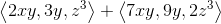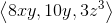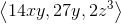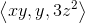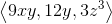Explanation:

The formula for adding two vectors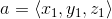and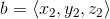is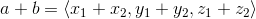. Using the vectors from the problem statement, this becomes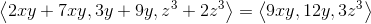### Example Question #39 : Vector Addition

Solve: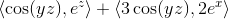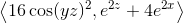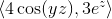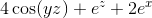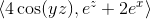Explanation:

To add two vectors, we simply add the corresponding components (for example,)### Example Question #40 : Vector Addition

Find the sum of the vectors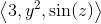and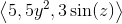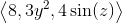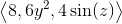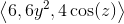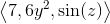To find the sum of two vectorsandis. Using the vectors we were given, we get